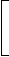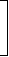# Aptitude - True Discount

Exercise : True Discount - General Questions
1.
A man purchased a cow for Rs. 3000 and sold it the same day for Rs. 3600, allowing the buyer a credit of 2 years. If the rate of interest be 10% per annum, then the man has a gain of:
0%
5%
7.5%
10%
Explanation:

C.P. = Rs. 3000.

 S.P. = Rs.3600 x 100= Rs. 3000. 100 + (10 x 2)

Gain = 0%.

2.
The true discount on Rs. 2562 due 4 months hence is Rs. 122. The rate percent is:
12%
 13 1 % 3
15%
14%
Explanation:

P.W. = Rs. (2562 - 122) = Rs. 2440.S.I. on Rs. 2440 for 4 months is Rs. 122.Rate =100 x 122% = 15%.
 2440 x 1 3

3.
A trader owes a merchant Rs. 10,028 due 1 year hence. The trader wants to settle the account after 3 months. If the rate of interest 12% per annum, how much cash should he pay?
Rs. 9025.20
Rs. 9200
Rs. 9600
Rs. 9560
Explanation:
Required money = P.W. of Rs. 10028 due 9 months hence
= Rs.10028 x 100100 +12 x 912
= Rs. 9200.

4.
A man wants to sell his scooter. There are two offers, one at Rs. 12,000 cash and the other a credit of Rs. 12,880 to be paid after 8 months, money being at 18% per annum. Which is the better offer?
Rs. 12,000 in cash
s. 12,880 at credit
Both are equally good
Explanation:
P.W. of Rs. 12,880 due 8 months hence
= Rs.12880 x 100100 +18 x 812
 = Rs.12880 x 100112
= Rs. 11500.

5.
If Rs. 10 be allowed as true discount on a bill of Rs. 110 due at the end of a certain time, then the discount allowed on the same sum due at the end of double the time is:
Rs. 20
Rs. 21.81
Rs. 22
Rs. 18.33
 T.D. on Rs. 110 = Rs.20 x 110= Rs. 18.33 120